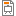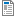# 17ABBFY1 - Physics I

Code Completion Credits Range Language
17ABBFY1 Z,ZK 5 2P+1S+1L English
Lecturer:
Tutor:
Supervisor:
Department of Natural Sciences
Synopsis:

Physics I course will allow students to acquire and strengthen knowledge in these branches of physics: mechanics, thermodynamics and solid state physics. We focus on solid theoretical bases, but independent work in student labs as well as solving practical examples are also important parts of the course. Through the course we also touch the limits of the classical Physics.

Requirements:

Assessment:

1. Completion of lab works and lab reports.

2. Completed all of seminars.

3. A grade of at least 60% in credit test.

Examination:

app. 100 minutes written exam, which includes both numerical exercises and theoretical questions.

Syllabus of lectures:

Outline of lectures:

1. Introduction, safety, physical measuremets, SI system of units and unit conversions.

2. Position of a particle, coordinate system, movements, vector quantities vs. scalar quantities and operations with them, average speed and instant speed, acceleration, uniformly accelerated motion, vertical throw.

3. Movement and force, momentum, equations of motion, Newton's laws, types of forces, composition of forces, momentum conversation law.

4. Work, energy, kinetic and potential energies, work of an elastic force, power, mechanical energy conservation law.

5. Gravitation field, gravitation force, Newton's law of universal gravitation, gravitation near Earth, potential energy of gravitation field, rockets and satellites, Kepler's laws.

6. Movements of bodies, Projectile motion, Friction force, Limit speed, Newton's formula, Stokes' formula, Circular motion. Equilibrium, Rotation of rigid bodies,

7. System of mass points, Center of gravity, First momentum law, Momentum and Impulse, Collisions - elastic and inelastic. Momentum, Moment of Inertia.

8. Oscillatory motion, Harmonic motion, Pendulums, Damped oscillator, Resonance.

9. Waves and their types, Travelling waves, Interference, Standing wave, Sound. Credit test.

10. Kinetic theory of matter, Molar quantities, Solid state matter - structure: description, deformations, elasticity, Hooke's law, Deformation curve.

11. Structure and properties of liquids, Pascal?s and Archimedes? laws, flow lines, Equation of continuity, Bernoulli´s equation.

12. Thermodynamics, Temperature, Heat, Internal energy, Heat transfer, Three thermodynamics laws, Kinetic theory of gases, Statistical description of phenomena in gases, Entropy.

Syllabus of tutorials:

Numerical exercises:

1) Kinematics and dynamics.

2) Kinetic energy, work, power, efficiency.

3) Gravitational field.

4) Mechanics of solids.

5) Liquids and gases, law of thermodynamics.

6) Oscillation and wave motions,credit test.

1) Moment of inertia.

2) Speed of sound.

3) Youngs moduli.

4) Kinematics and dynamics.

5) Ballistic curve.

6) Pendulum oscillation.

Study Objective:

Physics I will allow students to acquire and to strengthen a university-level knowledge in the following physics branches: mechanics, thermodynamics and solid state physics. The course is mainly focused on solid theoretical bases, but it also contains an important part of independent laboratory experiments and practical examples solving. Throughout the semester, this course reaches to the limits of classical physics.

Study materials:

 Halliday, D., Resnick, R., Walker, J. Fundamentals of Physics

Supplementary sources:

 Recorded video lectures and materials at MIT (Massachusetts Institute of Technology, USA), course „Classical Mechanics“,

http://ocw.mit.edu/courses/physics/8-01-physics-i-classical-mechanics-fall-1999/

 Feynman Lectures on Physics at California Institute of Technology: http://www.feynmanlectures.caltech.edu/

 Internet resources in the document „Internet Resources for Physics 1 and Physics 2“ published at the course website

Note:
The course is a part of the following study plans:

Lectures:
AttachmentSizeLecture 1200.85 KBLecture 2243.05 KBLecture 3491.08 KBLecture 4239.58 KBLecture 5918.11 KBLecture 61.2 MBLecture 71.72 MBLecture 82.64 MBLecture 9 and 102.77 MBLecture 111.79 MBLecture 121.4 MBExample of exam questions150.74 KB

Exercises:
AttachmentSizeExercise 1336.2 KBExercise 2461.64 KBSemestral test Example38.4 KBSolution values240.63 KBLabs 1 Newton’s second law Air track and Laws of collision v1.0431.05 KBLabs 2 Pendulum oscillations v0.9266.55 KBLabs 3 Projectile motion v0.9275.65 KBLabs 4 Vapour pressure of water below 100°C v1.0619.36 KBExercise 3461.36 KBExercise 4476.43 KBExercise 5491.99 KBExercise 6397.51 KBFinal exam Example150.97 KBLabs First page and structure50.58 KB

Others:
AttachmentSizeSchedule 17ABBFY1 - Physics I 20152016114.06 KBSchedule 17ABBFY1 - Physics I Exams 20152016104.11 KBSchedule 17ABBFY1 - Physics I Laboratory Exams 20152016106.5 KBSchedule 17ABBFY1 - Physics I Laboratory Exams 2016201713.36 KBSchedule 17ABBFY1 - Physics I Exams 2016201713.6 KBSchedule 17ABBFY1 - Physics I 20172018 seminars and laboratories29.3 KBSchedule 17ABBFY1 - Physics I 20172018 lectures273.4 KBTime table 1819_(P)_17ABBFY1387 KBTime table 1819_(C,L)_17ABBFY1374.3 KBSchedule b191-17abbfy1-lectures-201945.31 KBSchedule b191-17abbfy1-seminars -201943.13 KBSchedule b191-17abbfy1 laboratories -201943.18 KB